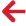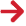﻿ PipeLay > Technical Notes > Technical Note 3 - Static Analysis Procedure

# Technical Note 3 - Static Analysis Procedure

Navigation:  PipeLay > Technical Notes >

# Technical Note 3 - Static Analysis ProcedureThis technical note describes the static analysis procedure performed automatically by PipeLay in order to statically analyse a pipeline installation configuration. The procedure undertaken depends on the model complexity.

(i)An initial static analysis is firstly performed to obtain an approximate initial configuration.

(ii)If Non-Linear Props in Quasi Static in the Project component Constants dialog is set to Yes then a non-linear static step is performed here where non-linear material properties are introduced before any quasi-static analyses are performed.

(iii)If the contact stiffness for certain supports has been omitted then a default optimum stiffness is introduced during this analysis stage.

(iv)If Optimise Buoyancy is specified for an articulated stinger then this optimised buoyancy is calculated and introduced here.

(v)A static offset analysis is performed to introduce any static offsets present in the model. For example, if you have specified any non-zero displacement terms when defining a Fixed Connection Point or a Vessel Connection Point in the Model component, any specified displacements are ignored in the first stage and applied here.

(vi)If installation criteria have been specified and the Multi-Pass option set to Yes, PipeLay endeavours to satisfy the criteria during this analysis stage with stable linear materials, otherwise this step is skipped. The model configuration is automatically adjusted in an iterative series of static analyses in order to obtain a configuration where the specified criteria are adhered to.

(vii)For models which contain non-linear materials, a static analysis is performed in which the material non-linearities are introduced. During previous analysis stages, all materials behave in a linear manner. This tends to reduce convergence difficulties associated with non-linear models.

(viii)For analyses which include a current profile, a static analysis is performed to introduce the current loading.

(ix)For models where Support Optimisation is specified, PipeLay will perform the optimisation in this analysis step to adjust support elevation to achieve the specified radius of curvature.

(x)If installation criteria have been specified with the Multi-Pass option set to Yes, a second pass criteria analysis is performed here with non-linear materials. If the Criteria – Multi-Pass option is set to No then this analysis will be the first and only criteria analysis. The multi-pass criteria analysis option should lead to a more robust solution as the bulk of criteria adjustments have been made with linear materials.

(xi)If you have included a sheave and a return cable in your model, PipeLay will automatically adjust the length of the return cable in order to achieve the specified sheave efficiency ratio.

(xii)If a default optimum stiffness is being used for certain supports then the value is refined here to account for any changes in loading introduced over the preceding stages. This refined value will then be passed to any subsequent dynamic analysis and so should improve run time performance.

(xiii)For models which incorporate a tensioner, the pipe is restrained at the vessel end during previous analysis stages. In this stage, the axial restraint at the vessel is removed and a non-linear tensioner (spring) element is inserted. The non-linear tensioner properties are adjusted to produce a restoring tension equal to the tension present at the vessel end in the previous stage.

(xiv)For models which include either seabed or support friction, a static analysis is performed to introduce the effects of friction.

(xv)If a dynamic analysis is requested with a start time greater than zero seconds, a final static analysis is carried out whose end time will coincide with the dynamic start time.